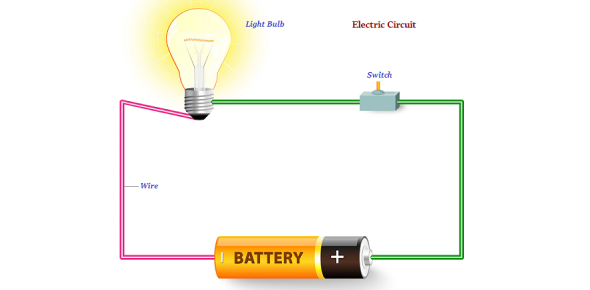# Physics- Light And Electricity Quiz

21 Questions | Total Attempts: 25SettingsAre you a physics student? If you are, then you must come to have across the topics of light and electricity. How much do you know about it? Well, this quiz has more than twenty questions, including MCQs, and T/Fs to let you test your knowledge of the topic. Let's see if you can pass this quiz with ease.

• 1.
Mixing green light with red light produces ................ light.
• A.

Cyan

• B.

Magenta

• C.

Yellow

• D.

Blue

• 2.
Objects can be seen clearly behind .............. material.
• A.

Opaque

• B.

Transparent

• C.

Translucent

• D.

Magnetic

• 3.
A black rock made of iron ores is called...
• A.

Magnesia

• B.

Magnetic force

• C.

Magnetite

• D.

Hematite

• 4.
The ability to attract materials is...
• A.

Non magnetic materials

• B.

Magnetic material

• C.

Magnetic field

• D.

Magnetic force

• 5.
When the light passes from air to water, it...
• A.

Splits into 7 spectrum colors

• B.

Changes its speed and direction

• C.

Reflect

• D.

• 6.
All of the following are magnetic materials except...
• A.

Copper

• B.

Steel

• C.

Iron

• D.

Cobalt

• E.

Nickel

• 7.
A set used to locate the four directions...
• A.

Electro-magnet

• B.

Compass

• C.

Dynamo

• D.

Prism

• 8.
Winch is one of the uses of...
• A.

Dynamo

• B.

Electromagnet

• C.

Prism

• D.

Compass

• 9.
A freely suspended magnet takes........................... direction.
• A.

North-west

• B.

East-west

• C.

North-south

• D.

West-south

• 10.
As we go from the two poles toward the center of the magnet, ............................
• A.

The attractive force increase

• B.

The magnetic force decrease

• C.

The magnetic force increase

• D.

No effect

• 11.
Mixing the blue, red and green light produce white light.
• A.

True

• B.

False

• 12.
A green apple is seen green through red glass sheet.
• A.

True

• B.

False

• 13.
Shadow is a darkened area which is formed as a result of falling of light on a transparent materials.
• A.

True

• B.

False

• 14.
Light can be separated into seven spectrum colors by using glass.
• A.

True

• B.

False

• 15.
The space around the magnet in which the effect of magnetic force appears is called magnetic field.
• A.

True

• B.

False

• 16.
A dynamo temporary magnet which is made by the effect of electricity.
• A.

True

• B.

False

• 17.
Magnetic force has electric effect and electric energy has magnetic effect.
• A.

True

• B.

False

• 18.
Dynamo is used to change magnetic energy into electric energy.
• A.

True

• B.

False

• 19.
Like poles attract each other.
• A.

True

• B.

False

• 20.
The idea of the camera depends on light travel in straight lines.
• A.

True

• B.

False

Related TopicsBack to top